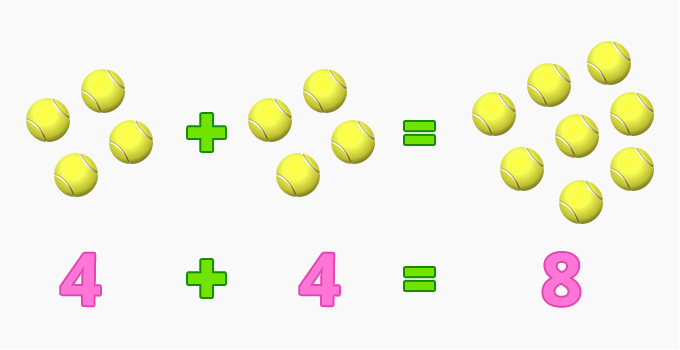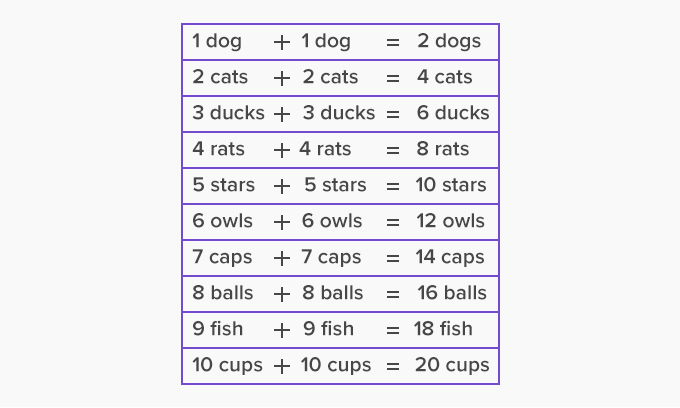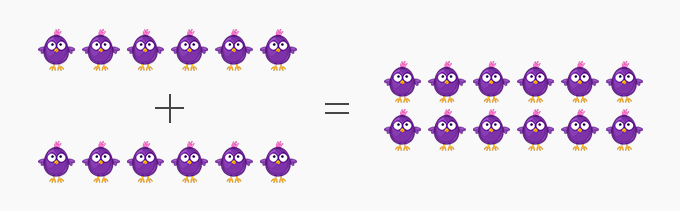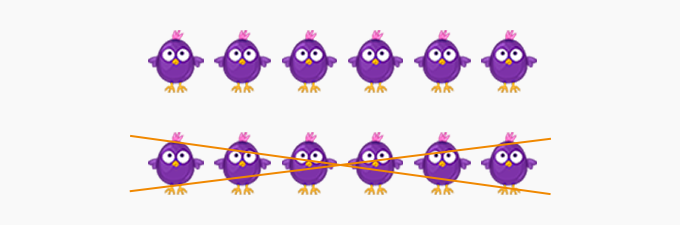# Doubles - Definition with Examples

The Complete K-5 Math Learning Program Built for Your Child

• 40 Million Kids

Loved by kids and parent worldwide

• 50,000 Schools

Trusted by teachers across schools

• Comprehensive Curriculum

Aligned to Common Core

## Doubles

To get a double of a number, we add the same number to itself. For example, double of 2 is 2  + 2 = 4.

Example: Michelle has 4 marbles and Jane has double the marbles that Michelle has. How many marbles does Jane have?

The double of 4 is 8.So, Jane has 8 marbles with her.

It is easy to remember the numbers that we get by doubling one-digit numbers.The addition of any two consecutive numbers can be done by using doubles plus 1 or doubles minus 1 strategy.

Example: 2 + 3

The number 3 is one more than 2. So, we can write 3 as 2 + 1. So, the addition 2 + 3 can be shown as:We already know the double of 2 is 4.

So, the required sum is one more than double. That is 5. Therefore, 2 + 3 = 5.

Example: 7 + 6

The number 6 is one less than 7. So, we can write 6 as 7 – 1. So, the addition 7 + 6 can be shown as:We already know the double of 7 is 14.

So, the required sum is one less than double. That is 13. Therefore, 7 + 6 = 13.

Doubles plus 1 and doubles minus 1 are also called near doubles strategy.

This can also be extended to the numbers that are not immediately next to each other.

Example: 5 + 8

8 is 3 more than 5.

5 + 8 = (5 + 5) + 3 = 10 + 3 = 13

## Doubles in subtraction:

An addend of an addition sentence will be the subtrahend/difference of the corresponding subtraction sentence.

We know that:

6 + 6 = 12

So, 12 – 6 = 6.

This can be pictorially shown as:If you take away 6 from 12:The remaining amount is 6.

 Fun Facts   Since 2 + 3 is the same as 3 + 2, we can apply any of the near doubles strategies to find the sum.    2 + 3 = (2 + 2) + 1 = 4 + 1 = 5                   OR    3 + 2 = (3 + 3 ) – 1 = 6 – 1 = 5

Won Numerous Awards & Honors